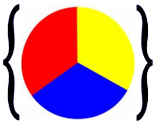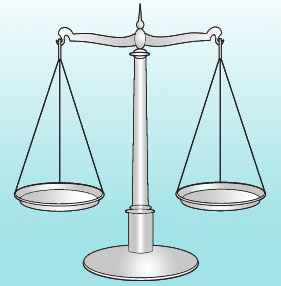# EqualityProblem 1: Mrs. Glosser asked her class to write the set of primary colors using roster notation. She received two different answers from two different students as shown below. Which student used the correct notation?

 Student Notation Eduardo X = {red, yellow, blue} Angie Y = {blue, red, yellow}

Solution: Both students used the correct notation.

The sets from problem 1 are equal, and we write X = Y. The equals sign (=) is used to show equality. Let's look at some more examples of set equality.

Example 1: Are sets A and B equal?

Solution:

 A = {1, 3, 5, 7} B = {3, 7, 1, 5}

Examine these sets closely to confirm that they are equal.

Since A and B contain exactly the same number of elements, and the elements in both are the same, we say that A is equal to B, and we write A = B. The order in which the elements appear in the set is not important.

Example 2: Are sets X and Y equal?

Solution:

 X = {a, e, i, o, u} Y = {u, o, i, e, a}

Examine these sets closely to confirm that they are equal.

Since X and Y contain exactly the same number of elements, we write X = Y. Remember that the order in which the elements appear in the set is not important.

Example 3: Are sets P and Q equal?

Solution:

 P = {apples, oranges, bananas, pears} Q = {oranges, pears, apples}

Examine these sets closely to confirm that they are not equal.

Since P and Q do not contain exactly the same elements, we say that P is not equal to Q, and we write P ≠ Q.

Example 4: Let R be the set of all whole numbers less than 5, and let S = {4, 2, 0, 3, 1}. Are sets R and S equal?

Solution:

 R = {whole numbers < 5} S = {4, 2, 0, 3, 1}

Examine these sets closely to confirm that they are equal.Example 5: Which of the following sets are equal?

 C = {1, 2, 3} D = {a, e, i, o, u} E = {2, 4, 6, 8, 10} F = {John, Jane, Joe} G = {2, 3, 1} H = {o, e, a, y, u} J = {2, 4, 6, 8} K = {Jane, Joe, John}

Answer: C = and F = K

In example 5, these sets are NOT equal: D ≠  H and E ≠ J. Can you name other sets that are not equal?

Summary: Two sets are equal if they have the exact same number of elements, and their elements are the same. The order in which the elements appear in the set is not important.